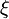# Reactive Processes

Performing material balances on reactive systems is slightly more complex than for non-reactive systems

N2 + 3H2 -> 2NH3

While we can still do a balance on total mass, this is often not particularly useful (as we would like to know component compositions). What differs now is that a balance on total moles will not work (in general).

While the book notes three types of reactive balances, there are really only two types that are clearly distinct: a molecular species/extent of reaction balance; and a balance on atomic species.

For simplicity, this course will only focus on reactive processes that are open systems which are run at steady state. This yields a simplified balance equation of:

0=MI−MO+G−C

Molecular/Extent of Reaction Balances

In this balance method we will examine each molecular species individually, and will typically rearrange our balance equation to be in the form:

MO=MI+G−C

The G and C terms will then come directly from a combination of stoichiometry and the concept of the extent of reaction.

DEFINITION

The extent of reaction () is a method of quantifying how many “times” a reaction has occurred. It has units of moles/time and numerically, it is chosen such that the stoichiometric coefficient timesis equal to the quantity of species reacted.

Using the above definition of the extent of reaction, it is a simple matter to write G and C in terms of this quantity simply using stoichiometry. In anticipation of performing reactive balances, we will switch to using molar quantities (n‘s rather than M‘s) so that we get:

nO=nI+g−c

where we have used lowercase g and c to note molar quantities.

EXAMPLE

Let’s write the expressions for our figure above:

NOTE:

Typically “reactants” have g=0, while “products” have a zero initial concentration and have c=0. This obviously becomes more complex when multiple reactions take place.

Using the extent of reaction method for systems with multiple reactions involves including a new value offor each reaction, and then calculating c and g as the sum of applicable‘s.

EXAMPLE

Consider the reaction network:

A -> B
2B -> C

If we assign1 to the first reaction and2 to the second, we get expressions that look like: